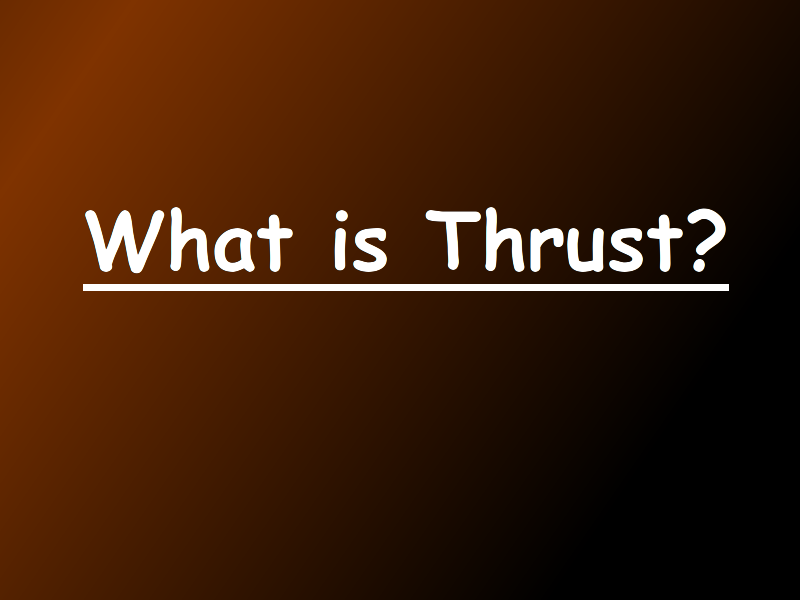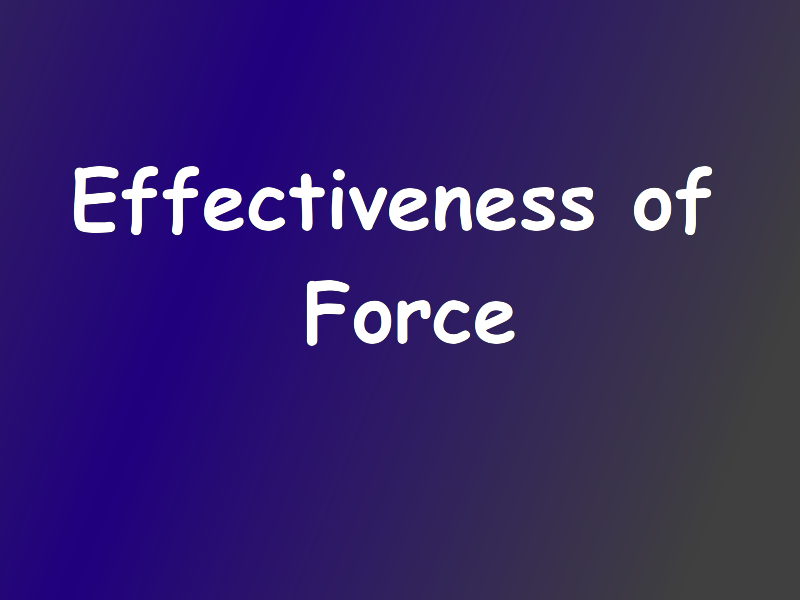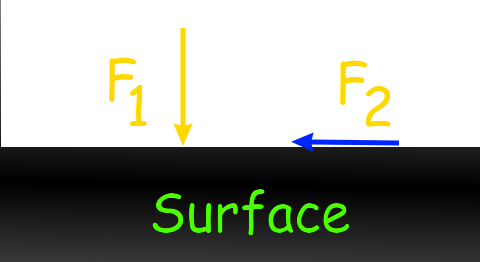Understanding Pressure

What is Force, Thrust, and Pressure? Atmospheric Pressure and Pressure applied by liquid.

Learner's Guide

Motivation:

In this session, we will find the answer to following questions:

• What is force?
• What is thrust?
• What is pressure?
• How does air exert pressure?
• How do liquids exert pressure?

------------------------------

What is Force?

1/7Effects of Force:

--------------------------------------

What is Thrust?

1/8What is the unit of Thrust?

1. Same as unit of force
2. Newton
3. Meter
4. Kilogram

Thrust is a type of force.

So unit of thrust is same as force.

Unit of Force = Unit of thrust = Newton

Correct answer: 1 & 2

Effectiveness of Force:

1/9Pressure: a measure of force's effectiveness:

If you apply more force, it will always be more effective.

True or False?

False.

Effectiveness of force depends on surface area and amount of force.

Pressure Exerted by Atmosphere and Liquids:

Why do we use mercury in barometer and thermometer?

• It is liquid at room temperature.
• It's characteristics do not change with change in pressure and temperature over a big range.
• For example: it behaves same at 10 ATM and at 1 ATM pressure.
• It behaves same at 100 degrees a 1 degree temperature.

Exercise

Tip: Think before answering.

•According to above image, Pressure on surface is:

1. F1/surface area
2. F1
3. F2
4. F2/Surface area
Difficulty Level: Moderate

F1/surface area

Pressure = Force acting perpendicular to surface / surface area.

• Effectiveness of force = _______________

1. Thrust
2. Perpendicular Force
3. Effective Force
4. Pressure
Difficulty Level: Easy

Pressure

• Pressure is measured by _______________

1. Manometer
2. Barometer
3. Thermometer
4. 1 & 2
Difficulty Level: Moderate

1 & 2

Barometer = Atmospheric Pressure

Manometer = Liquid Pressure

• Which of the following metal is used in barometer?

2. Mercury
3. Aluminium
4. Glass
Difficulty Level: Easy

Mercury

• Atmospheric pressure is caused by ____________

1. Weight of air
2. Motion of air particles
3. Both weight and motion
4. None
Difficulty Level: Moderate

Both weight and motion.

Gas particles are always in motion. Thus majority of pressure is contributed as they strike the surface.

• Liquid exert pressure because of _____________

1. Movement of particles
2. Weight
3. None
Difficulty Level: Easy

weight

• If we increase the surface area, pressure will decrease.

True or False.

Difficulty Level: Moderate

True.

If we increase surface area, same force will get divided and thus effectiveness of it will decrease.